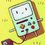# Synthetic Geometry Group - Alan Yan's Proposal

Note that although these questions are marked as "Synthetic", you are allowed to solve them with any method you want.

1. (Medium Difficulty) Let $ABC$ be a triangle and let $\omega$ be its incircle. Denote by $D_1$ and $E_1$ the points where $\omega$ is tangent to sides $BC$ and $AC$, respectively. Denote by $D_2$ and $E_2$ the points on sides BC and AC, respectively, such that $CD_2 = BD_1$ and $CE_2 = AE_1$, and denote by $P$ the point of intersection of segments $AD_2$ and $BE_2$. Circle $\omega$ intersects segment $AD_2$ at two points, the closer of which to the vertex A is denoted by Q. Prove that $AQ = D_2P$.

2. (Easy Difficulty) Let triangle $ABC$ satisfy $2BC = AB + AC$ and have incenter I and circumcircle $\omega$. Let D be the intersection of $AI$ and $\omega$ (with A, D distinct). Prove that I is the midpoint of AD.

3. (Hard Difficulty) Let the incircle $\omega$ of triangle $ABC$ touch $BC, CA,$ and $AB$ at $D , E$ and $F$, respectively. Let $Y_1 , Y_2, Z_1, Z_2$ and $M$ be the midpoints of $BF , BD, CE, CD$ and $BC$, respectively. Let the intersection of $Y_1Y_2$ and $Z_1Z_2$ be $X$. Prove that $MX \perp BC$.

If you want solutions posted, just comment down below.Note by Alan Yan
5 years, 10 months ago

This discussion board is a place to discuss our Daily Challenges and the math and science related to those challenges. Explanations are more than just a solution — they should explain the steps and thinking strategies that you used to obtain the solution. Comments should further the discussion of math and science.

When posting on Brilliant:

• Use the emojis to react to an explanation, whether you're congratulating a job well done , or just really confused .
• Ask specific questions about the challenge or the steps in somebody's explanation. Well-posed questions can add a lot to the discussion, but posting "I don't understand!" doesn't help anyone.
• Try to contribute something new to the discussion, whether it is an extension, generalization or other idea related to the challenge.

MarkdownAppears as
*italics* or _italics_ italics
**bold** or __bold__ bold
- bulleted- list
• bulleted
• list
1. numbered2. list
1. numbered
2. list
Note: you must add a full line of space before and after lists for them to show up correctly
paragraph 1paragraph 2

paragraph 1

paragraph 2

[example link](https://brilliant.org)example link
> This is a quote
This is a quote
    # I indented these lines
# 4 spaces, and now they show
# up as a code block.

print "hello world"
# I indented these lines
# 4 spaces, and now they show
# up as a code block.

print "hello world"
MathAppears as
Remember to wrap math in $$ ... $$ or $ ... $ to ensure proper formatting.
2 \times 3 $2 \times 3$
2^{34} $2^{34}$
a_{i-1} $a_{i-1}$
\frac{2}{3} $\frac{2}{3}$
\sqrt{2} $\sqrt{2}$
\sum_{i=1}^3 $\sum_{i=1}^3$
\sin \theta $\sin \theta$
\boxed{123} $\boxed{123}$

Sort by:

Q2. It is clear that $ABDC$ is cyclic. $\angle DBC = \angle DAC = \angle DAB = \angle DCB$. So, $\Delta DCB$ is isosceles and $DC = DB$. But, $\angle DIB = \angle DBI$(by some angle chasing). So, $DI = DB =DC$. But, since $ABDC$ is cyclic, using ptolemy's theorem we get $AD = 2DB = 2 DI$, which implies that $AI = ID$. So, $I$ is mid-point of $AD$.

- 5 years, 10 months ago

Does anyone want solutions?

- 5 years, 10 months ago

yes i want soutions please post the solutions.

- 5 years, 6 months ago